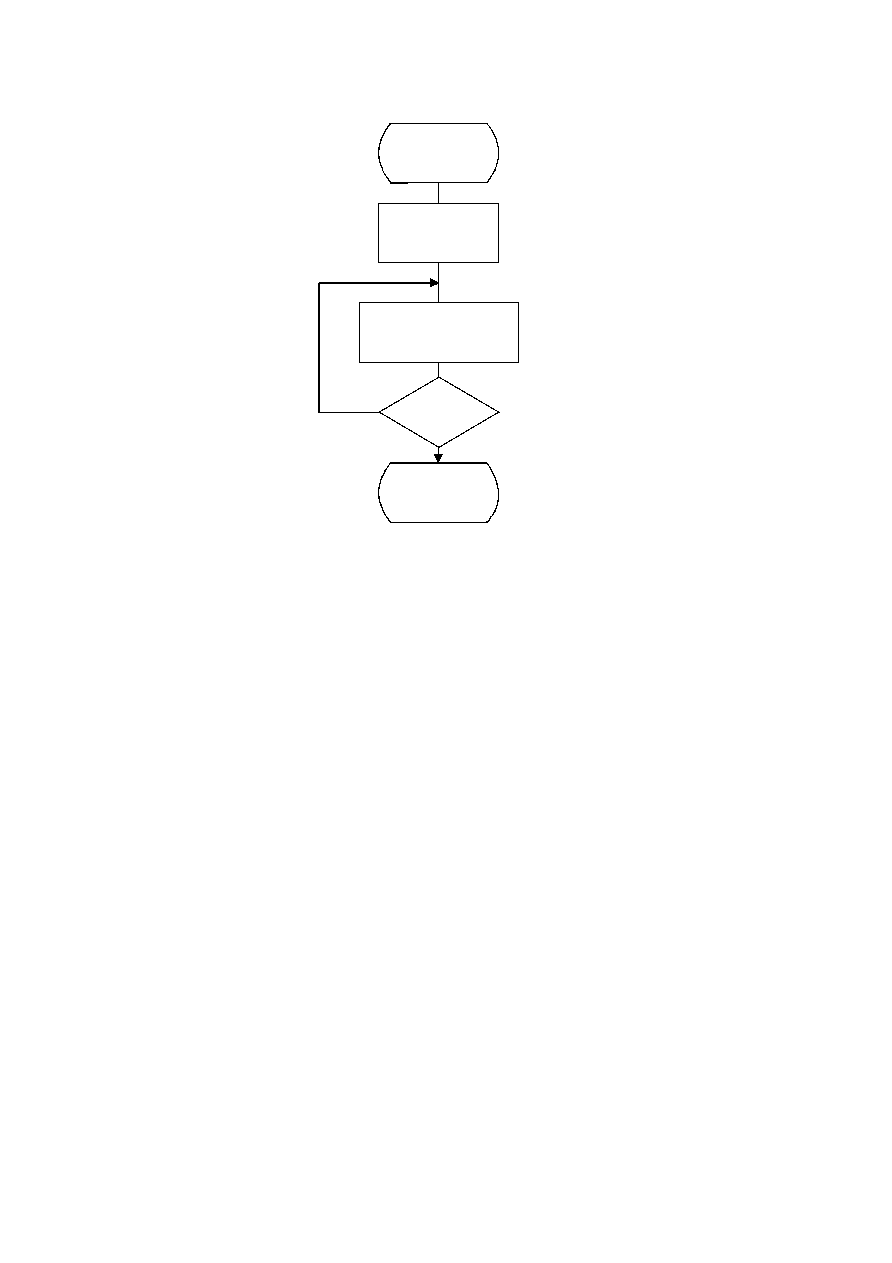ISO/IEC 10918-1 : 1993(E)
Figure C.3 illustrates this ordering procedure.
TISO1020-93/d038
Order_codes
K = 0
Yes
No
K < LASTK
?
Done
I = HUFFVAL(K)
EHUFCO(I) = HUFFCODE(K)
EHUFSI(I) = HUFFSIZE(K)
K = K + 1
Figure C.3 ­ Ordering procedure for encoding procedure code tables
Figure C.3 [D38], = 11.5 cm = 449.%
C.3
Bit ordering within bytes
The root of a Huffman code is placed toward the MSB (most-significant-bit) of the byte, and successive bits are placed in
the direction MSB to LSB (least-significant-bit) of the byte. Remaining bits, if any, go into the next byte following the
same rules.
Integers associated with Huffman codes are appended with the MSB adjacent to the LSB of the preceding Huffman code.
CCITT Rec. T.81 (1992 E)
53